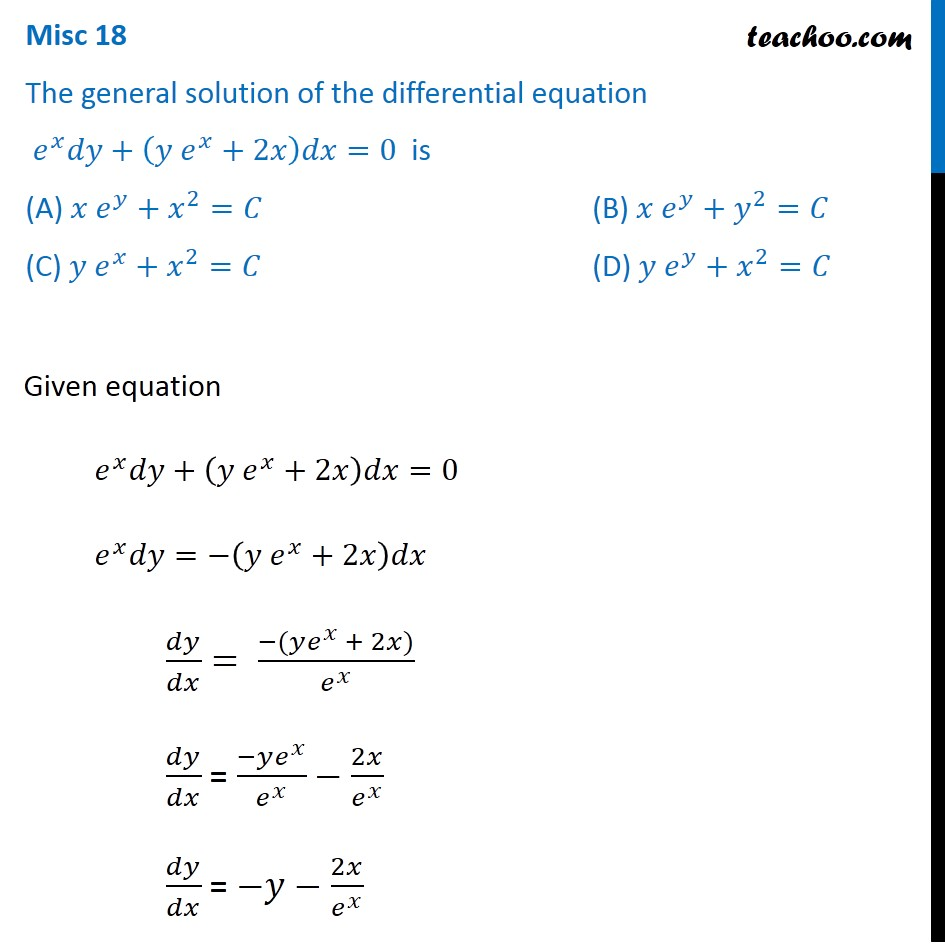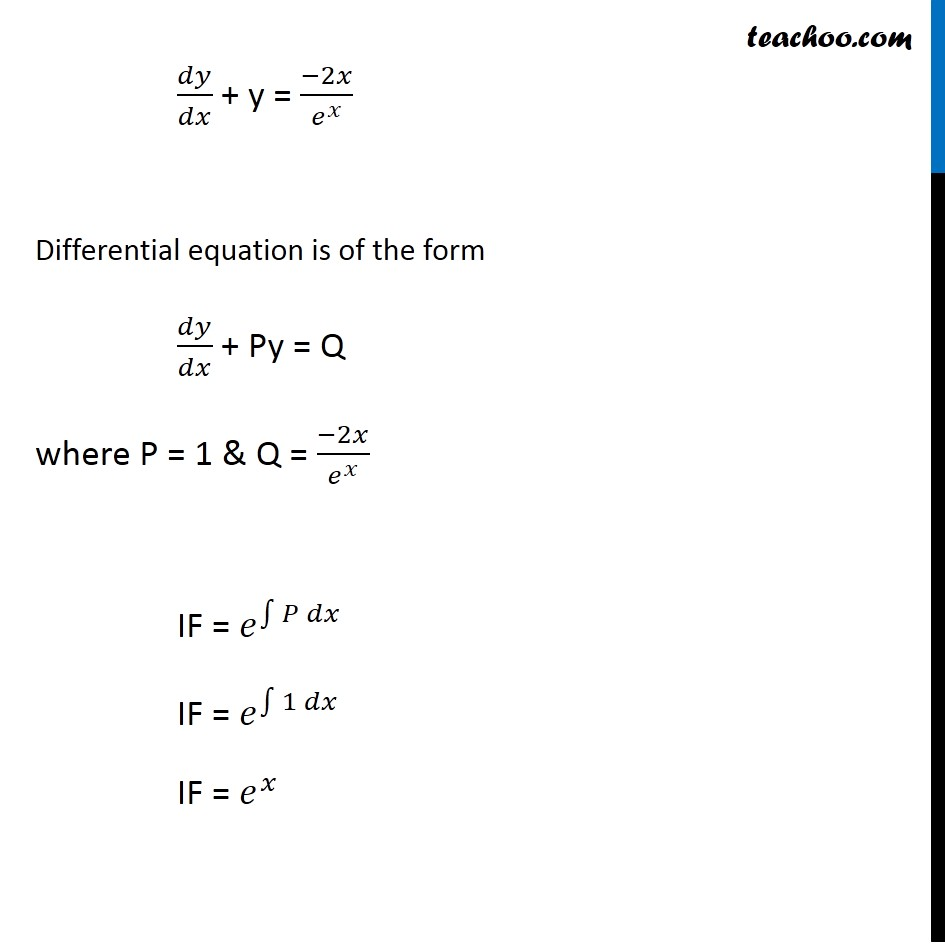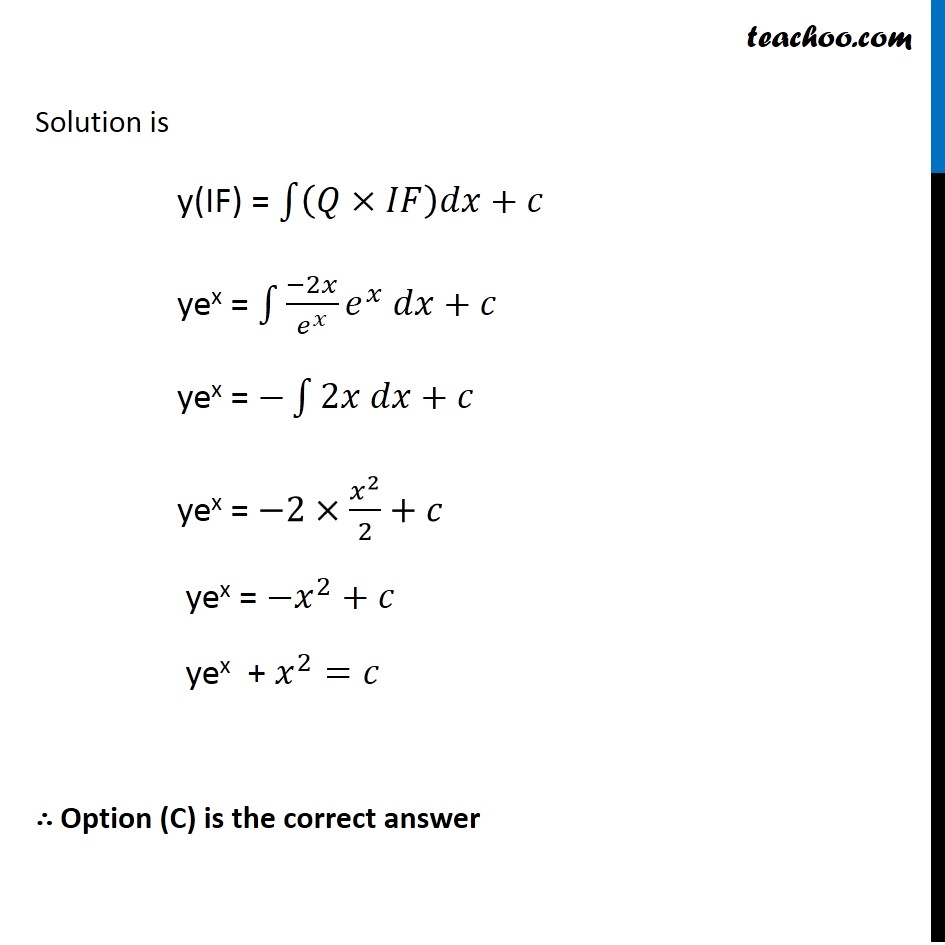Miscellaneous

Chapter 9 Class 12 Differential Equations
Serial order wiseLearn in your speed, with individual attention - Teachoo Maths 1-on-1 Class

### Transcript

Misc 18 The general solution of the differential equation 𝑒^𝑥 𝑑𝑦+(𝑦 𝑒^𝑥+2𝑥)𝑑𝑥=0 is (A) 𝑥 𝑒^𝑦+𝑥^2=𝐶 (B) 𝑥 𝑒^𝑦+𝑦^2=𝐶 (C) 𝑦 𝑒^𝑥+𝑥^2=𝐶 (D) 𝑦 𝑒^𝑦+𝑥^2=𝐶 Given equation 𝑒^𝑥 𝑑𝑦+(𝑦 𝑒^𝑥+2𝑥)𝑑𝑥=0 𝑒^𝑥 𝑑𝑦=−(𝑦 𝑒^𝑥+2𝑥)𝑑𝑥 𝑑𝑦/𝑑𝑥= (−(𝑦𝑒^𝑥 + 2𝑥))/𝑒^𝑥 𝑑𝑦/𝑑𝑥 = (−𝑦𝑒^𝑥)/𝑒^𝑥 −2𝑥/𝑒^𝑥 𝑑𝑦/𝑑𝑥 = −𝑦−2𝑥/𝑒^𝑥 𝑑𝑦/𝑑𝑥 + y = (−2𝑥)/𝑒^𝑥 Differential equation is of the form 𝑑𝑦/𝑑𝑥 + Py = Q where P = 1 & Q = (−2𝑥)/𝑒^𝑥 IF = 𝑒^∫1▒〖𝑃 𝑑𝑥〗 IF = 𝑒^∫1▒〖1 𝑑𝑥〗 IF = 𝑒^𝑥 Solution is y(IF) = ∫1▒〖(𝑄×𝐼𝐹)𝑑𝑥+𝑐〗 yex = ∫1▒〖(−2𝑥)/𝑒^𝑥 𝑒^𝑥 𝑑𝑥+𝑐〗 yex = −∫1▒〖2𝑥 𝑑𝑥+𝑐〗 yex = −2×𝑥^2/2+𝑐 yex = −𝑥^2+𝑐 yex + 𝑥^2=𝑐 ∴ Option (C) is the correct answer